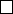7Transformation groups

IA Vectors and Matrices7.1 Groups of orthogonal matrices
Proposition.
The set of all
n × n
orthogonal matrices
P
forms a group under
matrix multiplication.
Proof.
0.
If
P, Q
are orthogonal, then consider
R
=
P Q
.
RR
T
= (
P Q
)(
P Q
)
T
=
P (QQ
T
)P
T
= P P
T
= I. So R is orthogonal.
1. I satisfies II
T
= I. So I is orthogonal and is an identity of the group.
2.
Inverse: if
P
is orthogonal, then
P
1
=
P
T
by definition, which is also
orthogonal.
3.
Matrix multiplication is associative since function composition is associative.
Definition
(Orthogonal group)
.
The orthogonal group
O
(
n
) is the group of
orthogonal matrices.
Definition
(Special orthogonal group)
.
The special orthogonal group is the
subgroup of O(n) that consists of all orthogonal matrices with determinant 1.
In general, we can show that any matrix in O(2) is of the form
cos θ sin θ
sin θ cos θ
or
cos θ sin θ
sin θ cos θ